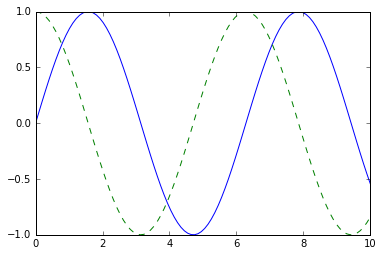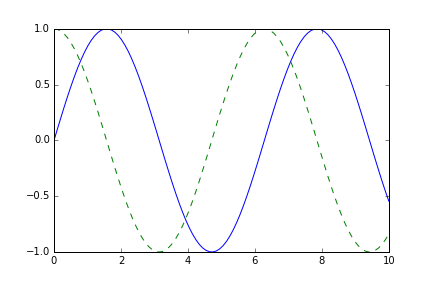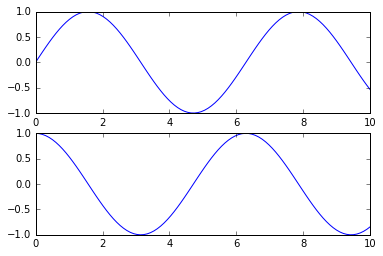8.3 Matplotlib 可视化

IPython 的创始人 Fernando Perez 当时正努力完成他的博士学位，并且 John 知道他几个月都没时间审查补丁。John 把它看做一个使自己开始的动机，之后 Matplotlib 软件包诞生了，2003 年发布了 0.1 版本。当它被用作太空望远镜科学研究所（哈勃望远镜背后的人）选择的绘图包时，它得到了早期的赞助，该研究所在财务上支持了 Matplotlib 的开发，并极大地扩展了它的能力。

Matplotlib 最重要的功能之一是，它能够很好地兼容许多操作系统和图形后端。Matplotlib 支持许多后端和输出类型，这意味着无论你使用哪种操作系统或你希望使用哪种输出格式，你都可以依赖它。

一般 Matplotlib 提示

导入 Matplotlib

import matplotlib as mpl
import matplotlib.pyplot as plt

设置样式

plt.style.use('classic')

show()还是不show()？如何展示你的绘图

来自脚本的绘图

# ------- 文件：myplot.py ------
import matplotlib.pyplot as plt
import numpy as np

x = np.linspace(0, 10, 100)

plt.plot(x, np.sin(x))
plt.plot(x, np.cos(x))

plt.show()

\$ python myplot.py

plt.show()命令做了很多工作，因为它必须与系统的交互式图形后端进行交互。此操作的细节可能因不同系统，甚至安装而异，但 matplotlib 会尽力对你隐藏所有这些细节。

来自 IPython shell 的绘图

In : %matplotlib
Using matplotlib backend: TkAgg

In : import matplotlib.pyplot as plt

来自 IPython 笔记本的绘图

IPython 笔记本是一个基于浏览器的，交互式数据分析工具，可以将叙述，代码，图形，HTML 元素等组合到一个可执行文档中（参见“IPython：不只是普通的 Python”）。

IPython 笔记本中的交互式绘图，可以使用%matplotlib命令完成，其工作方式与 IPython shell 类似。在 IPython 笔记本中，你还可以选择直接在笔记本中嵌入图形，有两种可能的选择：

• %matplotlib notebook将产生嵌入在笔记本中的交互式绘图
• %matplotlib inline将产生嵌入在笔记本中的绘图的静态图像

%matplotlib inline

import numpy as np
x = np.linspace(0, 10, 100)

fig = plt.figure()
plt.plot(x, np.sin(x), '-')
plt.plot(x, np.cos(x), '--');将图形保存到文件

Matplotlib 的一个很好的功能，是以各种格式保存图形的能力。保存图形可以使用savefig()命令完成。例如，要将上一个图形保存为 PNG 文件，可以运行以下命令：

fig.savefig('my_figure.png')

!ls -lh my_figure.png

# -rw-r--r--  1 jakevdp  staff    16K Aug 11 10:59 my_figure.png

from IPython.display import Image
Image('my_figure.png')savefig()中，文件格式是从给定文件名的扩展名推断出来的。根据你安装的后端，可以使用许多不同的文件格式。通过使用图形画布对象的以下方法，可以找到系统支持的文件类型列表：

fig.canvas.get_supported_filetypes()

'''
{'eps': 'Encapsulated Postscript',
'jpeg': 'Joint Photographic Experts Group',
'jpg': 'Joint Photographic Experts Group',
'pdf': 'Portable Document Format',
'pgf': 'PGF code for LaTeX',
'png': 'Portable Network Graphics',
'ps': 'Postscript',
'raw': 'Raw RGBA bitmap',
'rgba': 'Raw RGBA bitmap',
'svg': 'Scalable Vector Graphics',
'svgz': 'Scalable Vector Graphics',
'tif': 'Tagged Image File Format',
'tiff': 'Tagged Image File Format'}
'''

一个功能的两个接口

Matplotlib 的一个可能令人困惑的特性是它的两个接口：一个是方便的 MATLAB 风格的，基于状态的接口，以及一个更强大的面向对象的接口。我们将在这里快速强调两者之间的差异。

MATLAB 风格的接口

Matplotlib 最初编写为 MATLAB 用户的 Python 替代品，其大部分语法都反映了这一事实。MATLAB 风格的工具包含在pyplotplt）接口中。例如，以下代码可能对 MATLAB 用户来说非常熟悉：

plt.figure()  # 创建绘图图形

# 创建两个面板的第一个并设置当前轴
plt.subplot(2, 1, 1) # 行，列，面板号
plt.plot(x, np.sin(x))

# 创建第二个面板并设置当前轴
plt.subplot(2, 1, 2)
plt.plot(x, np.cos(x));面向对象的接口

# 首先创建绘图网格
# ax will be an array of two Axes objects
fig, ax = plt.subplots(2)

# 在对应对象上调用 plot() 方法
ax.plot(x, np.sin(x))
ax.plot(x, np.cos(x));